martedì 23 agosto 2016

Final Evaluation

Dear all,

GSoC16 is at the end, I want to thank you for giving me the opportunity to participate in this unique experience.

My goal was to add two solvers to the odepkg package, two exponential integrators, that are a class of numerical methods for the solution of partial and ordinary differential equations.

• Here is a summary of the work done in this months:

For the midterm evaluation (repository of the midterm work):

After a first period of study, I started with implementations.
I start with the implementation of the φ functions (phi1m.m, phi2m.m, phi3m.m, phi4m.m), necessary to calculate the matrix functions in the two schemes (exprk.m, exprb.m) for a general exponential Runge-Kutta and Rosenbrock integrator. These schemes have been very useful to verify the correctness of the official methods. I applied them to four different exponential methods (exprk2.m, exprk3.m, exprb3.m, exprb4.m).
Then I wrote a code that implements the augmented matrix  Ã (augmat.m) described in theorem 2.1 of [HAM 11]. This matrix will be given as input to expmv and will allow us to implement the exponential integrators by evaluating a single exponential of this augmented matrix avoiding the need to compute any φ functions.

For the final evaluation (REPOSITORY OF THE FINAL WORK):

After the midterm evaluation, I implemented the official exponential method that will be in odepkg, it is the advanced third order method with adaptive time stepping from Runge-Kutta family. Unlike the general schemes, for this code I used the function expmv so that I could evaluate a single exponential of an augmented matrix instead of computing any φ functions. I wrote a code for the stepper (exp_runge_kutta_23) and for the solver (odexprk23), according to odepkg standards.
Then I changed the file augmat.m to calculate a "double" augmented matrix, and in this way, I have been able to calculate, with a single call to expmv, both the solution to the next step, and the lower order solution for the estimation of the error.
In the same way I implemented the official exponential fourth order method with adaptive time stepping from Rosenbrock family (exp_rosenbrock_34, odexprb34)
I finally tested the order of my methods and I translate in C++ a for loop (__taylor__.cc) of the Nick Higham function expmv to improve the speed of an algorithm.

• In the repository of the final work you will find a patch for odepkg.

I added to the package the following files

/inst/odexprk23.m : Solve a set of stiff Ordinary Differential Equations (stiff ODEs) with a third-order exponential Runge-Kutta method.
/inst/odexprb34.m : Solve a set of stiff Ordinary Differential Equations (stiff ODEs) with a fourth-order exponential Rosenbrock method.
/inst/steppers/exp_runge_kutta_23.m : Stepper for the odexprk23 solver.
/inst/steppers/exp_rosenbrock_34.m : Stepper for the odexprb34 solver.
/inst/utilities/expmv.m : Compute the matrix exponential times vector or matrix (2010 Awad H. Al-Mohy and Nicholas J. Higham).
/inst/utilities/augmat.m, estimates.m, expmv_tspan.m, select_taylor_degree_aug.m, NormAm.m, select_taylor_degree.m, theta_taylor, theta_taylor_half, theta_taylor_single : Functions related to expmv.
/src/__taylor__.cc : for loop of expmv.

• I export the patch from
odepkg changeset: 542:3f74a6e911e1,
octave version: 4.1.0+, changeset: 22348:9deb86bb5632.
My patch exponential_integrators.diff requires a changeset >= 22345.
To apply the patch follow the commands

hg import exponential_integrators.diff
hg archive ../odepkg-0.9.1.tar.gz

run Octave 4.1.0+

pkg install odepkg-0.9.1.tar.gz

to test the patch:

demo odexprk23
demo odexprb34 (slightly slower)

if everything goes well, the demo gives a plot on convergence of methods.

•  Demos give the following warnings
warning: odexprk23: unknown field 'LinOp' in ODE options
warning: odexprk23: unknown field 'GFun' in ODE options

warning: odexprb34: unknown field 'DFdt' in ODE options
I haven't fixed the warnings because another GSoC16 student is working on the package and because outside the scope of my project, but the problem can quickly be solved collaborating at the official end of this experience.

martedì 26 luglio 2016

odexprb34 solver

As I did with the odexprk23 solver from the Runge-Kutta family, I implemented the official exponential fourth order method with adaptive time stepping from Rosenbrock family described in my postAlso in this case I used the function expmv and I wrote a code for the stepper (exp_rosenbrock_34), in which there are three calls to expmv function. The first two for the calculation of the second and third stage
$$U_{n2} = u_n + \frac{1}{2} h_n \varphi_1( \frac{1}{2} h_n J_n) F(t_n,u_n) + \frac{1}{2} h_n \varphi_2(\frac{1}{2} h_n J_n) \frac{1}{2} h_n v_n , \\ U_{n3} = u_n + h_n \varphi_1( h_n J_n) [F(t_n,u_n) + D_{n2}] + h_n \varphi_2 ( h_n J_n) h_n v_n ,$$
[Atilde, eta] = (1/2*dt, Jn, [Fn, 1/2*dt*vn]);
X = myexpmv (1/2*dt, Atilde, [zeros(size (Atilde)-1, 1); 1/eta])(1:d, :);

U(:, 2) = x(:) + X;

[Atilde, eta] = augmat (dt, Jn, [Fn+D(:, 2), dt*vn]);
X = myexpmv (dt, Atilde, [zeros(size (Atilde)-1, 1); 1/eta])(1:d, :);

U(:,3) = x(:) + X;

The third call to expmv is for both the solution to the next step, and the lower order solution for the estimation of the error

$$u_{n+1} = u_n + h_n \varphi_1(h_n J_n) F(t_n,u_n) + h_n \varphi_2(h_n J_n) h_n v_n \dots \\ \dots + h_n \varphi_3(h_n J_n) [ 16 D_{n2} - 2 D_{n3}] + h_n \varphi_4(h_n J_n) [ -48 D_{n2} + 12 D_{n3}] , \\ \hat{u}_{n+1} = u_n + h_n \varphi_1(h_n J_n) F(t_n,u_n) + h_n \varphi_2(h_n J_n) h_n v_n + h_n \varphi_3(h_n J_n) [ 16 D_{n2} - 2 D_{n3}] ,$$
v = [Fn, dt*vn, 16*D(:, 2)-2*D(:, 3), -48*D(:, 2)+12*D(:, 3)];
w = [Fn, dt*vn, 16*D(:, 2)-2*D(:, 3)];
[Atilde, eta] = augmat (dt, Jn, v, w);
p = size (v, 2);
q = size (w, 2);
ep = [[zeros(p-1, 1); 1/eta; zeros(q, 1)], [zeros(p+q-1, 1); 1/eta]];
X = [eye(d, d), zeros(d, p+q)]*myexpmv(dt, Atilde, [sparse(d, 1), sparse(d, 1); ep]);
X1 = X(:, 1);
X2 = X(:, 2);
x_next = x(:) + X1;
x_est = x(:) + X2;

I wrote also a code for the solver (odexprb34), according to odepkg standards and  I tested the order of the method with the usual example described in my post.

martedì 12 luglio 2016

odexprk23 solver

After the midterm evaluation, I implemented the official exponential method that will be in odepkg, it is the advanced third order method with adaptive time stepping from Runge-Kutta family described in my post. Unlike the general schemes, for this code I used the function expmv so that I could evaluate a single exponential of an augmented matrix instead of computing any φ functions. I wrote a code for the stepper (exp_runge_kutta_23), in which there are three calls to expmv function. The first two for the calculation of the second and third stage
$$U_{n2} = u_n + c_2 h_n \varphi_1(c_2 h_n A) F(t_n,u_n) , \\ U_{n3} = u_n + \frac{2}{3} h_n \varphi_1(\frac{2}{3} h_n A) F(t_n,u_n) + \frac{2}{3} h_n \varphi_2(\frac{2}{3} h_n A) \frac{2}{3 c_2}D_{n2} ,$$
myexpmv = @(t,A,v) expmv (t, A, v);

[Atilde, eta] = augmat (c2*dt, A, Fn);
X = myexpmv (c2*dt, Atilde, [zeros(size (Atilde)-1, 1); 1/eta])(1:d, :);

U(:, 2) = x(:) + X;

[Atilde, eta] = augmat (2/3*dt, A, [Fn, 2/(3*c2)*D(:, 2)]);
X = myexpmv (2/3*dt, Atilde, [zeros(size (Atilde)-1, 1); 1/eta])(1:d, :);

U(:,3) = x(:) + X;

Then I changed the file augmat.m to calculate a "double" augmented matrix, and in this way, I have been able to calculate, with a single call to expmv, both the solution to the next step, and the lower order solution for the estimation of the error

$$u_{n+1} = u_n + h_n \varphi_1(h_n A) F(t_n,u_n) + h_n \varphi_2(h_n A) \frac{3}{2}D_{n3} , \\ \hat{u}_{n+1} = u_n + h_n \varphi_1(h_n A) [ F(t_n,u_n) + \frac{1}{2 c_2}D_{n2}],$$
v = [Fn, 3/2*D(:, 3)];
w = [Fn + 1/(2*c2)*D(:, 2)];
[Atilde, eta] = augmat (dt, A, v, w);
p = size (v, 2);
q = size (w, 2);
ep = [[zeros(p-1, 1); 1/eta; zeros(q, 1)], [zeros(p+q-1, 1); 1/eta]];
X = [eye(d, d), zeros(d, p+q)]*myexpmv(dt, Atilde, [sparse(d, 1), sparse(d, 1); ep]);
X1 = X(:, 1);
X2 = X(:, 2);
x_next = x(:) + X1;
x_est = x(:) + X2;

I wrote also a code for the solver (odexprk23), according to odepkg standards.
I finally tested the order of the method with the usual example described in my post.

lunedì 20 giugno 2016

Week 4: augmented matrix

In this week I wrote a code that implements the augmented matrix described in theorem 2.1 of [HAM 11]. This matrix will be given as input to expmv and will allow us to implement the exponential integrators by evaluating a single exponential of this augmented matrix avoiding the need to compute any φ functions. The code is

function [Atilde, eta] = augmat(h,A,V)

p = size(V,2);
W = fliplr(V/diag(h.^(0:p-1)));
eta = 2^-ceil(log2(max(norm(W,1),realmin)));
Atilde = [ A , eta*W ; zeros(p,size(A)) , diag(ones(p-1,1),1) ];

in [HAM 11] eta is introduced to avoid rounding errors. I made a small change to avoid further errors, I added the max function so that smaller values ​​than realmin will not be considered.

Now I summarize my work during this month.

Week 1: phi functions. I implemented four functions, based on [BSW 07] (phi1m.m, phi2m.m, phi3m.m, phi4m.m).

Week 2-3: general exponential schemes. I implemented the two schemes for a general exponential Runge-Kutta and Rosenbrock integrator (exprk.m, exprb.m).
As already mentioned, these schemes are not really fast and efficient, but these are working codes that I applied to four different exponential methods (exprk2.m, exprk3.m, exprb3.m, exprb4.m) and I will use them as a reference when I go to implement the official methods.

Week 4: augmented matrix (augmat.m).

My codes can be found here

https://bitbucket.org/ChiaraSegala/chiara-segala

mercoledì 15 giugno 2016

Week 2-3: general exponential schemes

During these two weeks I implemented the two schemes for a general exponential Runge-Kutta and Rosenbrock integrator, see the schemes described in my second post.
I also implemented another file for exponential Runge-Kutta integrators based on the following scheme.
Given the problem
$$u'(t) = F(t,u) = A u(t) + g(t, u(t)), \\ u(t_0) = u_0,$$
the new scheme is
$$U_{ni} = u_n + c_i h_n \varphi_1(c_i h_n A) F(t_n,u_n) + h_n \sum_{j=2}^{i-1} a_{ij}(h_n A) D_{nj} , \\ u_{n+1} = u_n + h_n \varphi_1(h_n A) F(t_n,u_n) + h_n \sum_{i=2}^s b_i(h_n A) D_{ni} ,$$
where
$$D_{nj} = g(t_n + c_j h_n, U_{nj}) - g(t_n, u_n).$$
The main motivation for this reformulation is that the vectors $D_{nj}$ are expected to be small in norm so the application of matrix functions to these vectors are more efficient.

I applied the general pattern to the third-order exponential Runge-Kutta method with tableau

where $\varphi_{j,k} =\varphi_j (c_k h A)$ and $\varphi_j =\varphi_j (h A)$.

Then I also applied the general Rosenbrock pattern to the fourth-order exponential method with a third-order error estimator. Its coefficients are

where $\varphi_j =\varphi_j (h J_n)$.

I tested the correctness and order of the schemes with the following example, a semilinear parabolic problem
$$\frac{\partial U}{\partial t} (x,t) - \frac{\partial^2 U}{\partial x^2} (x,t) = \frac{1}{1 + U(x,t)^2} + \Phi (x,t)$$
for $x \in [0,1]$ and $t \in [0,1]$ with homogeneous Dirichlet boundary conditions. $\Phi$ is chosen in such a way that the exact solution of the problem is $U(x,t) = x(1-x)e^{t}$. I discretize this problem in space by standard finite differences with 200 grid points.

For details see [HO 10] and [HO 05].

Within this week I will insert on Bitbucket the codes of phi functions and the three general schemes .

mercoledì 1 giugno 2016

Week 1: phi functions

During the first week of GSoC, I wrote four m-files for matrix functions, phi1m,..., phi4m.
I implemented the four functions, based on [BSW 07].
Matrix functions are defined by the recurrence relation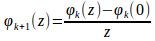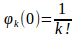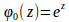In my files, I use an algorithm based on first a variant of the scaling and squaring approach and after scaling, I use a Padé approximation.
Below the code phi1m.m

function [N, PHI0] = phi1m (A, d)

N is φ1(A) and PHIO is φ0(A). d is the order of the Padé approximation.
First, I scale A by power of 2 so that its norm is < ½

l=1;

s= min (ceil (max (0,1+log2 (norm (A, inf)))), 1023);

A = A/2^s;

then I use a (d,d)-Padé approximation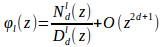where the polynomials are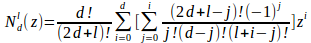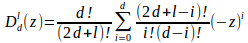I write polynomials in Horner form.

ID = eye (size (A));

i=d;

Ncoeff = sum (cumprod ([1, d-(0:i-1)])./cumprod ([1, 2*d+l-(0:i-1)]).*(-1).^(0:i)./(factorial ((0:i)).*factorial (l+i-(0:i))));

Dcoeff = ((-1)^(i))*prod ([(d-i+1):1:d])/(prod ([(2*d+l-i+1):1:(2*d+l)])*factorial (i));

N= Ncoeff;

D= Dcoeff;

for i = (d-1):-1:0

Ncoeff = sum (cumprod ([1, d-(0:i-1)])./cumprod ([1, 2*d+l-(0:i-1)]).*(-1).^(0:i)./(factorial ((0:i)).*factorial (l+i-(0:i))));

N = A*N + Ncoeff * ID ;

Dcoeff = ((-1)^(i))* prod([(d-i+1):1:d])/(prod ([(2*d+l-i+1):1:(2*d+l)])*factorial (i));

D = A*D + Dcoeff * ID ;

endfor

N = full (D\N);

and finally I use the scaling relations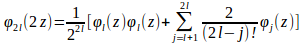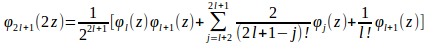PHI0 = A*N+ID;

for i = 1:s

N = (PHI0+ID)*N/2;

PHI0 = PHI0*PHI0;

endfor

in the same way I wrote the other three files.  As soon as possible, I will create a repository on Bitbucket and I will put the codes there.

sabato 14 maggio 2016

Phi functions and general exponential schemes

Hello!

May 23 will start the coding period and I'm trying to figure out how I will implement my functions before the mid-term evaluation.

With the advice of my mentors Marco and Jacopo, I decided to start with the implementation of the phi functions, necessary to calculate the matrix functions in the two schemes for a general exponential Runge-Kutta and Rosenbrock integrator.
These schemes will not be really fast and efficient, but I will use them as a reference when I go to implement the official methods. It will be useful to verify the correctness of my codes.

As regards the implementation of the phi functions I will refer to

[BSW 07] “EXPINT — A MATLAB Package for Exponential Integrators”, Havard Berland, Bard Skaflestad and Will M. Wright, 2007,
DOI: 10.1145/1206040.1206044, webpage (software without a license).

While the general schemes that then I'm going to implement are as follows:

• Exponential Runge-Kutta integrators
Consider a problem of the form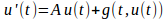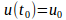,

the numerical exponential Runge Kutta scheme for its solution is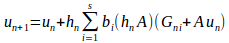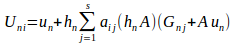where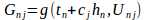and the coefficients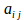and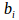are constructed from exponential functions or approximations of such functions.

• Exponential Rosenbrock integrators
Consider a problem of the form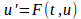,

the numerical exponential Rosenbrock scheme for its solution is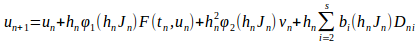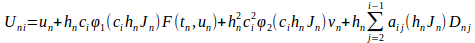where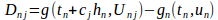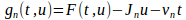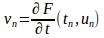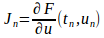for details about formulas see [HO 10].